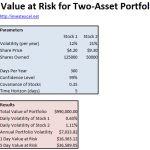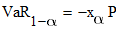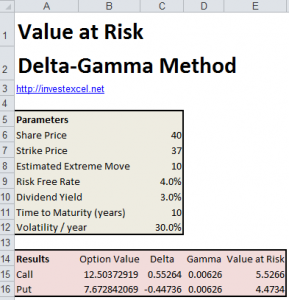# Value at Risk – Methods and Free Spreadsheets

This article summarizes several methods of calculating Value at Risk, and provides pricing spreadsheets. Value at Risk is an important tool for estimating capital requirements, and is now a standard risk-management tool.

Two parameters define the nature of Value at Risk. These are the confidence level (often 95% or 99%), and the holding period (often a day or a month). Value at Risk is simply the greatest expected loss over the holding period at the given confidence level.

## Variance-Covariance Method

This approach for calculating the value at risk is also known as the delta-normal method. It needs the average returns, variances and correlation coefficients (derived from historical data). The variance-covariance method assumes that historical returns are normally distributed, and that the future will mirror the past.The calculation is straightforward, and for a one-asset portfolio is given by this equation.xα is the αth percentile of a normal distribution, and P is the portfolio value. xα is calculated with Excel’s NORM.S.INV() function. The equations for a two-asset portfolio are given here

## Historical Simulation

This method employs historical returns data to assemble the cumulative distribution function, and does not place any assumptions on the shape of the distribution.

A historical simulation simply sorts the returns by size.  If the sample include 100 returns, the value at risk at a confidence of 95% is the fifth largest loss.

Several criticisms are often made of this approach.

• Historical simulation assumes that returns are independent and identically distributed.  This not necessarily the case; real-world data often displays volatility clustering.
• Returns in the recent-past and far-past are given equal weighting. However, recent returns have greater bearing on future behavior than older returns.
• This method requires a large set of historical data for accuracy; this, however, is not always available.
• Because the method is entirely reliant on historical data, the result cannot be influenced by subjective information (as with Monte-Carlo simulation). This may be significant if a fund manager predicts large changes in the business environment.

The variance-covariance approach can only be used for portfolios with a linear relationship between investment weights and risk. However, options have nonlinear payoffs, which becomes significant close to expiry. Standard variance-covariance methods cannot necessarily be applied.

Quadratic methods (also known as delta-gamma methods) were developed to estimate the Value at Risk for portfolios with options.## Monte-Carlo Method

The Monte-Carlo Method involves running multiple trials to calculate the portfolio returns.  Generally, this method involves the following steps.

• Generate simulated returns by sampling a probability distribution.
• Order the returns by size
• Compute the VaR at the required confidence level. For a simulation of 1000 returns, the 95% percentile would correspond to the 50th lowest value.

Monte-Carlo simulation is an extremely flexible method for calculating Value at Risk. This is because any probability distribution can be selected for all the significant risk factors. However, for a large investment universe, Monte-Carlo simulation can be computationally intensive.

This spreadsheet uses VBA for the Monte-Carlo simulation of VaR. It assumes a normal distribution, but can be adapted to any other distribution.### 4 thoughts on “Value at Risk – Methods and Free Spreadsheets”

1. Hi! I found the contents of this page very very informative and the spreadsheets are excellent. However, the historical simulation spreadsheet is not available, but I understood the methodology.

Suppose, my portfolio includes 4 stocks, 3 bonds, 4 forex transactions and say 2 derivative instruments (say 2 options). How do I find the the Value at Risk using say Var covar or Monte carlo simulation. I understand this is very general question and I just need general methodology. I shall be very grateful to you if you can guide me. I tried to search lot about portfolio var, but so far I am yet to come across some methodology. Perhaps, I am to be blamed for that.

Regards

Katherine

2. Hi,

Very useful. Thanks. Your formula for arriving at Annual Portfolio Volatility of \$7033.82 uses daily volatility of each stock. So wondering if the computation is ‘Daily’ PF volatility instead of ‘Annual’ PF volatility. Do correct me if I am wrong.

Regards